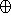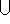## CGAL::offset_polygon_2

#include <CGAL/offset_polygon_2.h>

template<class ConicTraits, class Container>
typename Gps_traits_2<ConicTraits>::Polygon_with_holes_2
 offset_polygon_2 ( Polygon_2 pgn, typename ConicTraits::Rat_kernel::FT r, ConicTraits traits)
Computes the offset of the given polygon P by a given radius r - namely, the function computes the Minkowski sum PBr, where Br is a disc of radius r centered at the origin. Note that as the input polygon not be convex, its offset may not be a simple polygon. The result is therefore represented as a generalized polygon with holes, such that the edges of the polygon correspond to line segment and circular arcs, both are special types of conic arcs, as represented by the traits class.
 Precondition: P is a simple polygon.

template<class ConicTraits, class Container, class DecompositionStrategy>
typename Gps_traits_2<ConicTraits>::Polygon_with_holes_2
 offset_polygon_2 ( Polygon_2 pgn, typename ConicTraits::Rat_kernel::FT r, DecompositionStrategy decomp, ConicTraits traits)
Computes the exact representation of the offset of the given polygon P by a radius r, as described above. If P is not convex, the function decomposes it into convex sub-polygons P1, ..., Pk and computes the union of sub-offsets (namelyi(PiBr)). The decomposition is performed using the given decomposition strategy decomp, which must be an instance of a class that models the concept PolygonConvexDecomposition.
 Precondition: P is a simple polygon.